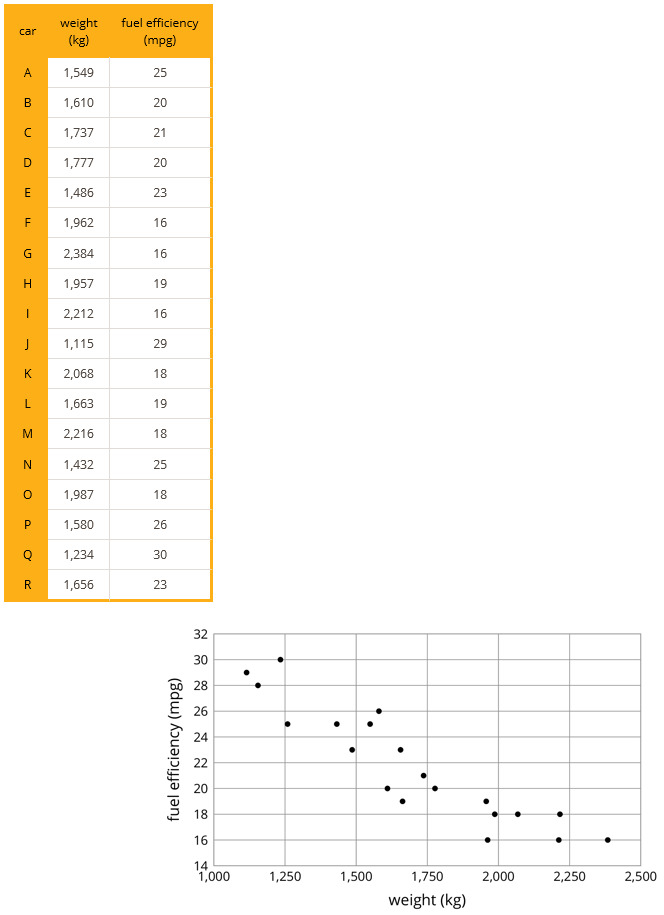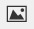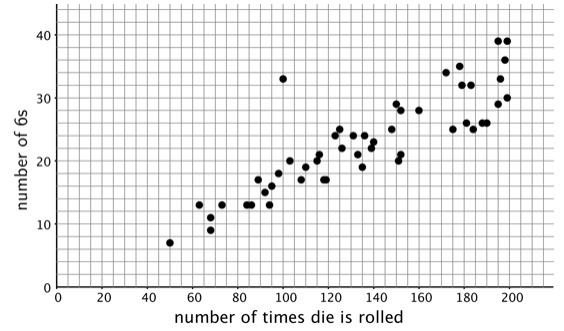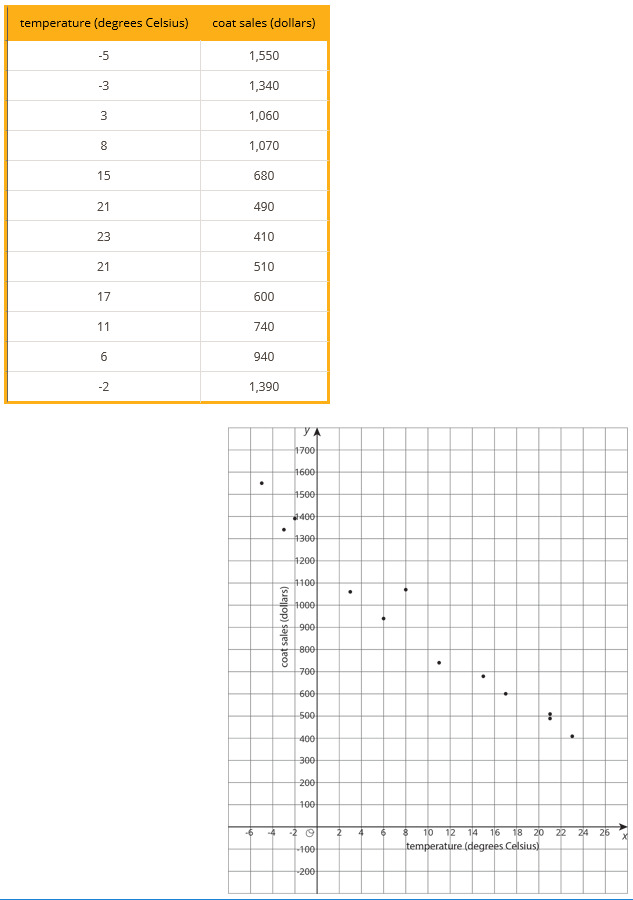PSABM6WQ - Student Facing Task---Acc7.5 Lesson 18 What a Point in a Scatter Plot Means (5.G.A.2, 8.AP.A.1, 8.AP.3)

A giant panda lives in a zoo.Giant Panda 2 Copyright Owner: Sheila Lau (Own Work) License: Public DomainWhat does the point on the graph tell you about the panda?

Part A)

The table and scatter plot show weights and fuel efficiencies of 18 cars.Which point in the scatter plot represents Car L's measurements?

(x,y)

with no spaces.

Part B)

What is the fuel efficiency of the car with the greatest weight?

Type your answer below as a number (example: 5, 3.1, 4 1/2, or 3/2):
Part C)

What is the weight of the car with the greatest fuel efficiency?

Type your answer below as a number (example: 5, 3.1, 4 1/2, or 3/2):
Part D)

Car S weighs 1,912 kilograms and gets 16 miles per gallon. On the scatter plot, plot a point that represents Car S’s measurements.

Draw your graph on paper, take a picture, and upload it using the upload iconIf you do not have the ability to upload an image of your work, type "Graph is on paper."

Part E)

Cars N and O, shown in the scatter plot, are made by the same company. Compare their weights and fuel efficiencies. Does anything surprise you about these cars?

Part F)

A different company makes Cars F and G. Compare their weights and fuel efficiencies. Does anything surprise you about these cars?

After a board game competition, the tournament director collects 50 dice from the games played and rolls each one until he gets bored and tries a different one. The scatter plot shows the number of times he rolled each die and the number of 6s that resulted during those rolls.Select a point in the scatter plot and give its approximate coordinates, then tell the story of that point in the context of the problem.

Part A)

A clothing store keeps track of the average monthly temperature in degrees Celsius and coat sales in dollars.What does the point (15, 680) represent?

Part B)

For the month with the lowest average temperature, estimate the total amount made from coat sales.

Type your answer below as a number (example: 5, 3.1, 4 1/2, or 3/2):
Part C)

Explain how you used the table to find this information.

Part D)

For the month with the smallest coat sales, estimate the average monthly temperature.

Type your answer below as a number (example: 5, 3.1, 4 1/2, or 3/2):
Part E)

Explain how you used the scatter plot to find this information.

Part F)

If there were a point at (0,A) what would it represent? Use the scatter plot to estimate a value for A.

Part G)

What would a point at (B,0) represent? Use the scatter plot to estimate a value for B.